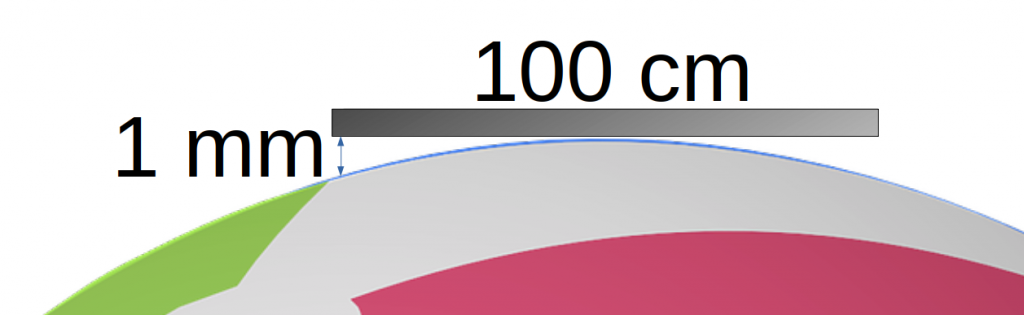# When would the rule test work?

A video of a guy defending the Earth’s flatness circulates on the internet, based on the argument that we cannot perceive the curvature when observing its horizon, ‘not even with the aid of a ruler’. The purpose of this post however is not to criticize your argument, but to show which planet dimension your argument would be enough to see the curvature on the horizon through this method.

In the video, they mention a ‘monstrous’ scale, but due to the information reported in the video itself, he says he is in Magé (RJ) and can see the municipalities of Duque de Caxias and São Gonçalo (both RJ) at both ends of his visual field. Putting it on a map, we have that its observable horizon should look something like the image below.

Based on this illustration, we have a viewing angle of 96 degrees, and a radius of approximately 14km (rounding upwards). With that, we have that the arc of the horizon of this observer must be (also rounding upwards) of 24 km, while the straight line that joins the vertices of this arc would have a distance (rounded upwards) of 21 km.

Let’s say that the ruler used is 1 m long, completely covers the entire 21 km of its linear visual field and the boy has a perfectly tangent alignment of the ruler with the horizon at a position of 50 cm (very favorable conditions for the boy).

To make it clearer what we will do, imagine that the Earth is one of those plastic balls with a radius of 50 cm, and we place our ruler tangent to its top. We have an unevenness from one end of the ruler to its curvature of 50 cm.

Thus, as we are using the 21 km visual field scale, we would have that the sphere above would have a radius of 10.5 km and an unevenness at the ends of the 10.5 km rule. What we are going to do now is to increase the size of our ball until we reach the smallest unevenness noticeable by the person who performs this experiment (say 1 mm from the ruler, which would be the equivalent of 21 m).Representation of the idea of ​​what we will do (but it is not yet on the correct scale).

Now redrawing a right triangle with a vertex in the center of the ball, we would have a figure as shown below, in which the hypotenuse and the leg have a difference of ‘a’ units of measurement, in this case, 1 mm or 21 m if we consider the scale.

We have that the hypotenuse of this triangle h, will be the radius of the Earth, the largest leg C, will be the radius of the Earth minus 21 meters and the smallest leg will be 105000 meters.

Thus, cos(theta) = (h-21)/h, sin(theta) = 10500/h and tang(theta) = 10500/(h-21). Making the trigonometric arcs of these three functions, and making them equal (since the angles theta and hypotenuse h are fixed), we arrive that h must be equal to 2,625,010.5 m. That is, a planet with a maximum radius of 2,625.0105 km.

We arrived thus, that its surface area of ​​this planet should have a maximum of 86,590,840 km².

Comparatively, the area of ​​the Asian continent is 44,580,000 km² and the area of ​​the American continent is 42,550,000 km², both continents together would occupy 87,130,000 km², that is, slightly more than 100% of the area of ​​one planet on which the ruler test would work.

With that, we concluded that the test of the screen would in fact be able to identify the curvature when observing the horizon, since our planet was much smaller than it really is.